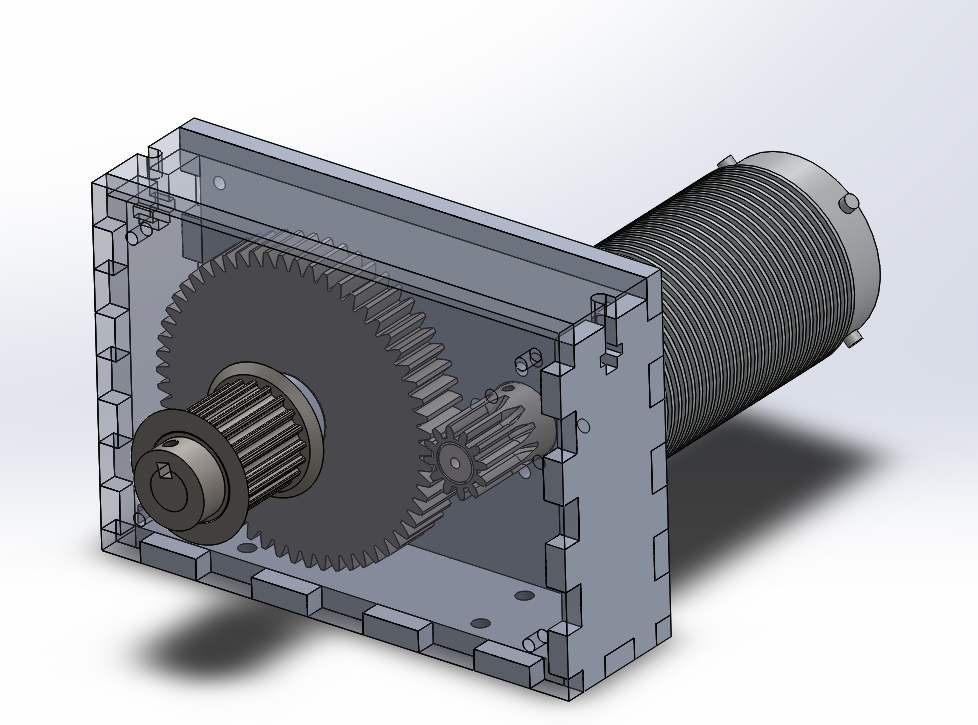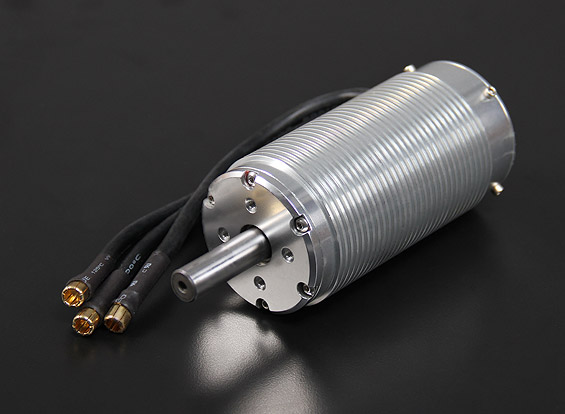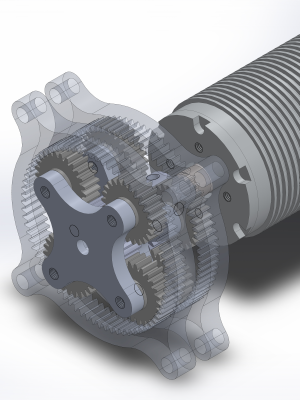It seems like a rite of passage for many MIT engineering students to make their own powered vehicle to get across campus quickly.

I had these goals in mind:

• Be capable of going 35 miles per hour on a flat surface (not on the streets, though)
• Be as light as possible (use 80/20 aluminum extrusion for the frame)
• Have 3 wheels

So, to begin this ritual, in October, I purchased this:Turnigy Trackstar 1/5 Sensorless BLDC

Now, if you look at the spec sheet for this motor, you come across a spec called Kv. This number specifies that maximum RPM per volt at which the motor will run. If you also look at the spec sheet you will also see that the motor is rated for 30 volts maximum, which gives a maximum RPM of around 22,000!

The downside to this is that although the motor will run really fast, the Kv of a motor is the inverse of the Kt of the motor which gives us:

$(\frac{760\cdot2 \pi}{\mathrm{V\cdot min}})^{-1} = 0.013\frac{\mathrm{N\cdot m}}{\mathrm{A}}$

Which times the maximum rated current (150 amps) gives us

$0.013\frac{\mathrm{N\cdot m}}{\mathrm{A}}\cdot 150:\mathrm{A} = 1.885:\mathrm{N\cdot m}$

That isn't a lot of torque, and since I'm using 8 inch wheels and if I were to direct drive I would only produce about 4 pounds of force at maximum current.

But wait! I said I wanted my maximum speed to be about 35 miles per hour (which is pretty fast when you're low), so I can find the gear ratio I need.

$\frac{22,000}{\text{min}}\div\frac{35\text{ mph}}{\pi\cdot 8\text{ in}} \approx \frac{22,000\text{ min}^{-1}}{1470\text{ min}^{-1}}\approx 15:1$

That's a high ratio.

So what do you do? You either design or buy a gearbox that can reduce the speed of the motor and generate the massive amounts of torque necessary.

So, what did I do? I designed two gearboxes: a planetary gearbox, and a more traditional gearbox.Now, in actuality, the voltage I chose to use with the motor was a nominal 39.8 volts, or 12 A123 LiFePO$_\text{4}$ batteries in series, so the gear ratio is about 20:1.